In :
import numpy as np
import pandas as pd
import seaborn as sns
from matplotlib import pyplot as plt

from sklearn.preprocessing import StandardScaler
from sklearn.model_selection import train_test_split, GridSearchCV, StratifiedKFold, \
learning_curve, validation_curve
from sklearn.linear_model import LogisticRegression
from sklearn.metrics import accuracy_score, confusion_matrix, precision_score, recall_score
from sklearn.decomposition import PCA

from scipy.stats import mannwhitneyu

sns.set(font_scale=1.5)
pd.options.display.max_columns = 50


## Project plan¶

1. Feature and data explanation
1. Primary data analysis
1. Primary visual data analysis
1. Insights and found dependencies
1. Metrics selection
1. Model selection
1. Data preprocessing
1. Cross-validation and adjustment of model hyperparameters
1. Creation of new features and description of this process
1. Plotting training and validation curves
1. Prediction for test or hold-out samples
1. Conclusions

## 1. Feature and data explanation¶

In :
df = pd.read_csv('data.csv')

In :
df.head()

Out:
id diagnosis radius_mean texture_mean perimeter_mean area_mean smoothness_mean compactness_mean concavity_mean concave points_mean symmetry_mean fractal_dimension_mean radius_se texture_se perimeter_se area_se smoothness_se compactness_se concavity_se concave points_se symmetry_se fractal_dimension_se radius_worst texture_worst perimeter_worst area_worst smoothness_worst compactness_worst concavity_worst concave points_worst symmetry_worst fractal_dimension_worst Unnamed: 32
0 842302 M 17.99 10.38 122.80 1001.0 0.11840 0.27760 0.3001 0.14710 0.2419 0.07871 1.0950 0.9053 8.589 153.40 0.006399 0.04904 0.05373 0.01587 0.03003 0.006193 25.38 17.33 184.60 2019.0 0.1622 0.6656 0.7119 0.2654 0.4601 0.11890 NaN
1 842517 M 20.57 17.77 132.90 1326.0 0.08474 0.07864 0.0869 0.07017 0.1812 0.05667 0.5435 0.7339 3.398 74.08 0.005225 0.01308 0.01860 0.01340 0.01389 0.003532 24.99 23.41 158.80 1956.0 0.1238 0.1866 0.2416 0.1860 0.2750 0.08902 NaN
2 84300903 M 19.69 21.25 130.00 1203.0 0.10960 0.15990 0.1974 0.12790 0.2069 0.05999 0.7456 0.7869 4.585 94.03 0.006150 0.04006 0.03832 0.02058 0.02250 0.004571 23.57 25.53 152.50 1709.0 0.1444 0.4245 0.4504 0.2430 0.3613 0.08758 NaN
3 84348301 M 11.42 20.38 77.58 386.1 0.14250 0.28390 0.2414 0.10520 0.2597 0.09744 0.4956 1.1560 3.445 27.23 0.009110 0.07458 0.05661 0.01867 0.05963 0.009208 14.91 26.50 98.87 567.7 0.2098 0.8663 0.6869 0.2575 0.6638 0.17300 NaN
4 84358402 M 20.29 14.34 135.10 1297.0 0.10030 0.13280 0.1980 0.10430 0.1809 0.05883 0.7572 0.7813 5.438 94.44 0.011490 0.02461 0.05688 0.01885 0.01756 0.005115 22.54 16.67 152.20 1575.0 0.1374 0.2050 0.4000 0.1625 0.2364 0.07678 NaN

### 1.1 Process of collecting data¶

Features are computed from a digitized image of a fine needle aspirate (FNA) of a breast mass. They describe characteristics of the cell nuclei present in the image. n the 3-dimensional space is that described in: [K. P. Bennett and O. L. Mangasarian: "Robust Linear Programming Discrimination of Two Linearly Inseparable Sets", Optimization Methods and Software 1, 1992, 23-34].

The data used is available through https://www.kaggle.com/uciml/breast-cancer-wisconsin-data
And can be found on UCI Machine Learning Repository: https://archive.ics.uci.edu/ml/datasets/Breast+Cancer+Wisconsin+%28Diagnostic%29
This database is also available through the UW CS ftp server: ftp ftp.cs.wisc.edu cd math-prog/cpo-dataset/machine-learn/WDBC/

### 1.2 Detailed explanation of the task¶

The task here is to predict whether the cancer is benign or malignant based in 30 real-valued features.

### 1.3 Target features¶

Attribute Information:

1) ID number
2) Diagnosis (M = malignant, B = benign)
3-32)

Ten real-valued features are computed for each cell nucleus:
a) radius (mean of distances from center to points on the perimeter)
b) texture (standard deviation of gray-scale values)
c) perimeter
d) area
e) smoothness (local variation in radius lengths)
f) compactness (perimeter^2 / area - 1.0)
g) concavity (severity of concave portions of the contour)
h) concave points (number of concave portions of the contour)
i) symmetry
j) fractal dimension ("coastline approximation" - 1)

The mean, standard error and "worst" or largest (mean of the three largest values) of these features were computed for each image, resulting in 30 features. For instance, field 3 is Mean Radius, field 13 is Radius SE, field 23 is Worst Radius.

All feature values are recoded with four significant digits.
Missing attribute values: none
Class distribution: 357 benign, 212 malignant

## 2. Primary data analysis¶

### 2.0 Data preprocessing¶

In :
target = pd.DataFrame(df['diagnosis'])
data = df.drop(['diagnosis'], axis=1)


### 2.1 Constant columns¶

General data overview:

In :
data.info()

<class 'pandas.core.frame.DataFrame'>
RangeIndex: 569 entries, 0 to 568
Data columns (total 32 columns):
id                         569 non-null int64
texture_mean               569 non-null float64
perimeter_mean             569 non-null float64
area_mean                  569 non-null float64
smoothness_mean            569 non-null float64
compactness_mean           569 non-null float64
concavity_mean             569 non-null float64
concave points_mean        569 non-null float64
symmetry_mean              569 non-null float64
fractal_dimension_mean     569 non-null float64
texture_se                 569 non-null float64
perimeter_se               569 non-null float64
area_se                    569 non-null float64
smoothness_se              569 non-null float64
compactness_se             569 non-null float64
concavity_se               569 non-null float64
concave points_se          569 non-null float64
symmetry_se                569 non-null float64
fractal_dimension_se       569 non-null float64
texture_worst              569 non-null float64
perimeter_worst            569 non-null float64
area_worst                 569 non-null float64
smoothness_worst           569 non-null float64
compactness_worst          569 non-null float64
concavity_worst            569 non-null float64
concave points_worst       569 non-null float64
symmetry_worst             569 non-null float64
fractal_dimension_worst    569 non-null float64
Unnamed: 32                0 non-null float64
dtypes: float64(31), int64(1)
memory usage: 142.3 KB


Drop constant column Unnamed: 32 and id column which is useless for analize:

In :
data.drop(['Unnamed: 32', 'id'], axis=1, inplace=True)


### 2.2 Missing values¶

Check data for missing values:

In :
print("Are there missing values:", data.isnull().values.any())

Are there missing values: False


### 2.3 Summary statistics¶

General data statistics overview:

In :
data.describe()

Out:
radius_mean texture_mean perimeter_mean area_mean smoothness_mean compactness_mean concavity_mean concave points_mean symmetry_mean fractal_dimension_mean radius_se texture_se perimeter_se area_se smoothness_se compactness_se concavity_se concave points_se symmetry_se fractal_dimension_se radius_worst texture_worst perimeter_worst area_worst smoothness_worst compactness_worst concavity_worst concave points_worst symmetry_worst fractal_dimension_worst
count 569.000000 569.000000 569.000000 569.000000 569.000000 569.000000 569.000000 569.000000 569.000000 569.000000 569.000000 569.000000 569.000000 569.000000 569.000000 569.000000 569.000000 569.000000 569.000000 569.000000 569.000000 569.000000 569.000000 569.000000 569.000000 569.000000 569.000000 569.000000 569.000000 569.000000
mean 14.127292 19.289649 91.969033 654.889104 0.096360 0.104341 0.088799 0.048919 0.181162 0.062798 0.405172 1.216853 2.866059 40.337079 0.007041 0.025478 0.031894 0.011796 0.020542 0.003795 16.269190 25.677223 107.261213 880.583128 0.132369 0.254265 0.272188 0.114606 0.290076 0.083946
std 3.524049 4.301036 24.298981 351.914129 0.014064 0.052813 0.079720 0.038803 0.027414 0.007060 0.277313 0.551648 2.021855 45.491006 0.003003 0.017908 0.030186 0.006170 0.008266 0.002646 4.833242 6.146258 33.602542 569.356993 0.022832 0.157336 0.208624 0.065732 0.061867 0.018061
min 6.981000 9.710000 43.790000 143.500000 0.052630 0.019380 0.000000 0.000000 0.106000 0.049960 0.111500 0.360200 0.757000 6.802000 0.001713 0.002252 0.000000 0.000000 0.007882 0.000895 7.930000 12.020000 50.410000 185.200000 0.071170 0.027290 0.000000 0.000000 0.156500 0.055040
25% 11.700000 16.170000 75.170000 420.300000 0.086370 0.064920 0.029560 0.020310 0.161900 0.057700 0.232400 0.833900 1.606000 17.850000 0.005169 0.013080 0.015090 0.007638 0.015160 0.002248 13.010000 21.080000 84.110000 515.300000 0.116600 0.147200 0.114500 0.064930 0.250400 0.071460
50% 13.370000 18.840000 86.240000 551.100000 0.095870 0.092630 0.061540 0.033500 0.179200 0.061540 0.324200 1.108000 2.287000 24.530000 0.006380 0.020450 0.025890 0.010930 0.018730 0.003187 14.970000 25.410000 97.660000 686.500000 0.131300 0.211900 0.226700 0.099930 0.282200 0.080040
75% 15.780000 21.800000 104.100000 782.700000 0.105300 0.130400 0.130700 0.074000 0.195700 0.066120 0.478900 1.474000 3.357000 45.190000 0.008146 0.032450 0.042050 0.014710 0.023480 0.004558 18.790000 29.720000 125.400000 1084.000000 0.146000 0.339100 0.382900 0.161400 0.317900 0.092080
max 28.110000 39.280000 188.500000 2501.000000 0.163400 0.345400 0.426800 0.201200 0.304000 0.097440 2.873000 4.885000 21.980000 542.200000 0.031130 0.135400 0.396000 0.052790 0.078950 0.029840 36.040000 49.540000 251.200000 4254.000000 0.222600 1.058000 1.252000 0.291000 0.663800 0.207500

Conclusion: here we can see vary different min/max values for features, for example area_mean and smoothness_mean. Thus we should check for outliers (box plot is good option for that).

### 2.4 Statistics for different classes¶

Check if difference of features mean values is statistically important. We will use Mann Whitney criteria, because of it unsesetive to outliers and different samples distribution.

In :
for column in data.columns:
m = data[column][target['diagnosis']=='M']
b = data[column][target['diagnosis']=='B']
statistic, pvalue = mannwhitneyu(m, b)

print('Column:', column, 'Important:', pvalue < 0.05 )

Column: radius_mean Important: True
Column: texture_mean Important: True
Column: perimeter_mean Important: True
Column: area_mean Important: True
Column: smoothness_mean Important: True
Column: compactness_mean Important: True
Column: concavity_mean Important: True
Column: concave points_mean Important: True
Column: symmetry_mean Important: True
Column: fractal_dimension_mean Important: False
Column: texture_se Important: False
Column: perimeter_se Important: True
Column: area_se Important: True
Column: smoothness_se Important: False
Column: compactness_se Important: True
Column: concavity_se Important: True
Column: concave points_se Important: True
Column: symmetry_se Important: True
Column: fractal_dimension_se Important: True
Column: texture_worst Important: True
Column: perimeter_worst Important: True
Column: area_worst Important: True
Column: smoothness_worst Important: True
Column: compactness_worst Important: True
Column: concavity_worst Important: True
Column: concave points_worst Important: True
Column: symmetry_worst Important: True
Column: fractal_dimension_worst Important: True


Conclusion: differences in almost all features are statistically important. So they will contribute more enough information to classification.

### 2.5 Target feature¶

Number of eamples for each class:

In :
target['diagnosis'].value_counts()

Out:
B    357
M    212
Name: diagnosis, dtype: int64

Let's check the ratio of examples belong to each class:

In :
target['diagnosis'].value_counts() / target['diagnosis'].size

Out:
B    0.627417
M    0.372583
Name: diagnosis, dtype: float64

Conclusion: there are a lot more examples for benign class, but not enough for skewed classes problem.

## 3. Primary visual data analysis¶

For the sake of informative data visualization we need to standardize and scale features, because of some features have very different max/min values.

In :
scaler = StandardScaler()
scaled_data = scaler.fit_transform(data)
data_scaled = pd.DataFrame(scaled_data, columns=data.columns)
data_scaled['diagnosis'] = target['diagnosis']


### 3.1 Linear dependecies of the features (correlation matrix):¶

Helper function for plotting feature correlations:

In :
def plot_corr(data):
plt.figure(figsize=[40, 40])
ax = sns.heatmap(data.corr(), annot=True, fmt= '.1f', linewidths=.5)
ax.set_xticklabels(ax.get_xticklabels(), size='xx-large')
ax.set_yticklabels(ax.get_yticklabels(), size='xx-large')
plt.show();


Data correlations:

In :
plot_corr(data)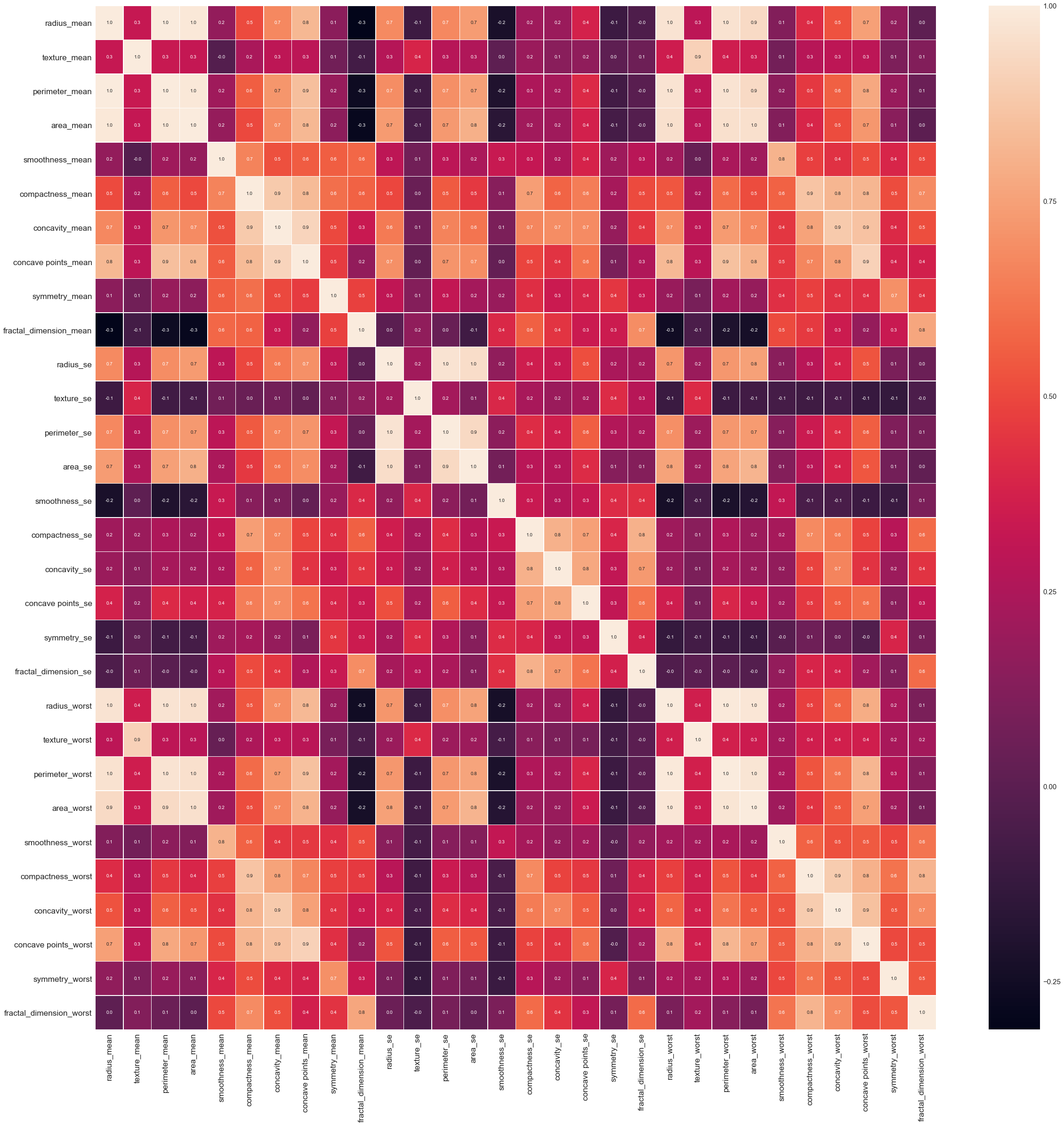Conclusion: there are several groups of correlated features:

• compactness_mean, concavity_mean, concave points_mean
• compactness_worst, concavity_worst, concave points_worst
• compactness_se, concavity_se, concave points_se
• texture_mean, texture_worst
• area_worst, area_mean

### 3.2 Outliers¶

In :
data_z = pd.melt(data_scaled, id_vars="diagnosis", var_name="features", value_name='value')

In :
plt.figure(figsize=(20, 10));
ax = sns.boxplot(x='features', y='value', hue='diagnosis', data=data_z);
ax.set_xticklabels(ax.get_xticklabels());
plt.xticks(rotation=90);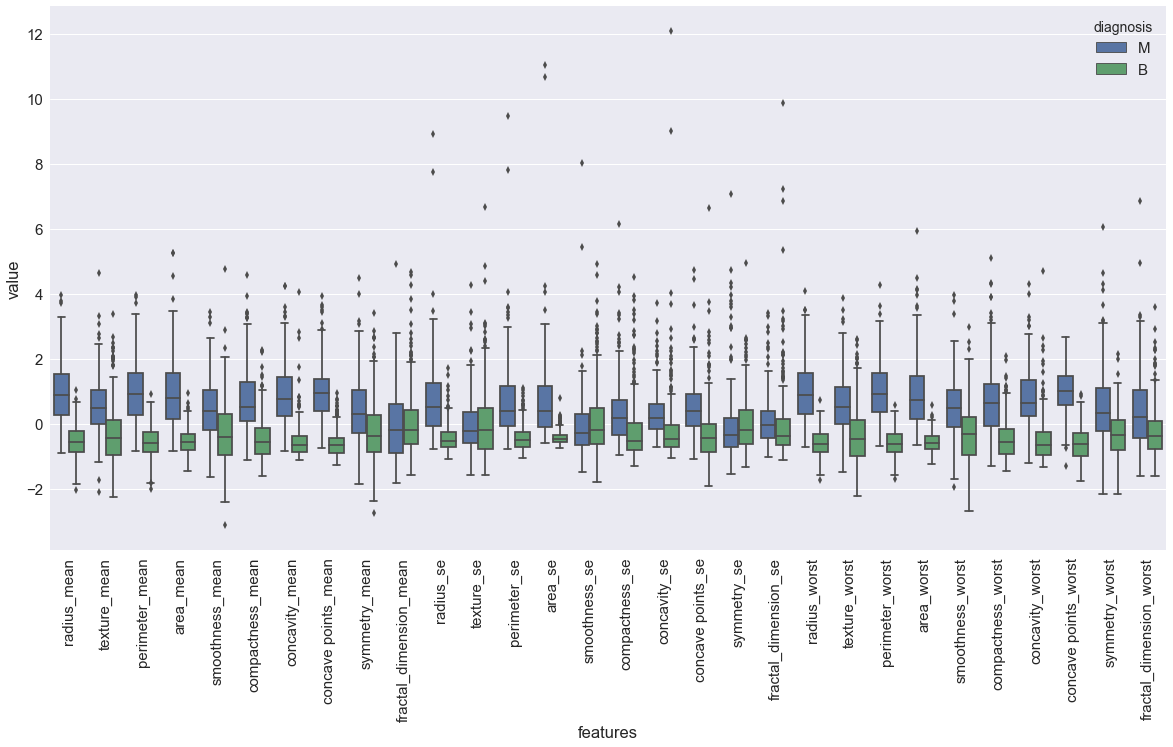Conclusion: there are a lot of variable with outliers. So before training we have to handle it.

### 3.3 Distribution of classes¶

In :
plt.figure(figsize=(30, 20));
ax = sns.violinplot(x="features", y="value", hue="diagnosis", data=data_z, split=True, inner="quartile");
ax.set_xticklabels(ax.get_xticklabels(), size='large');
plt.xticks(rotation=90);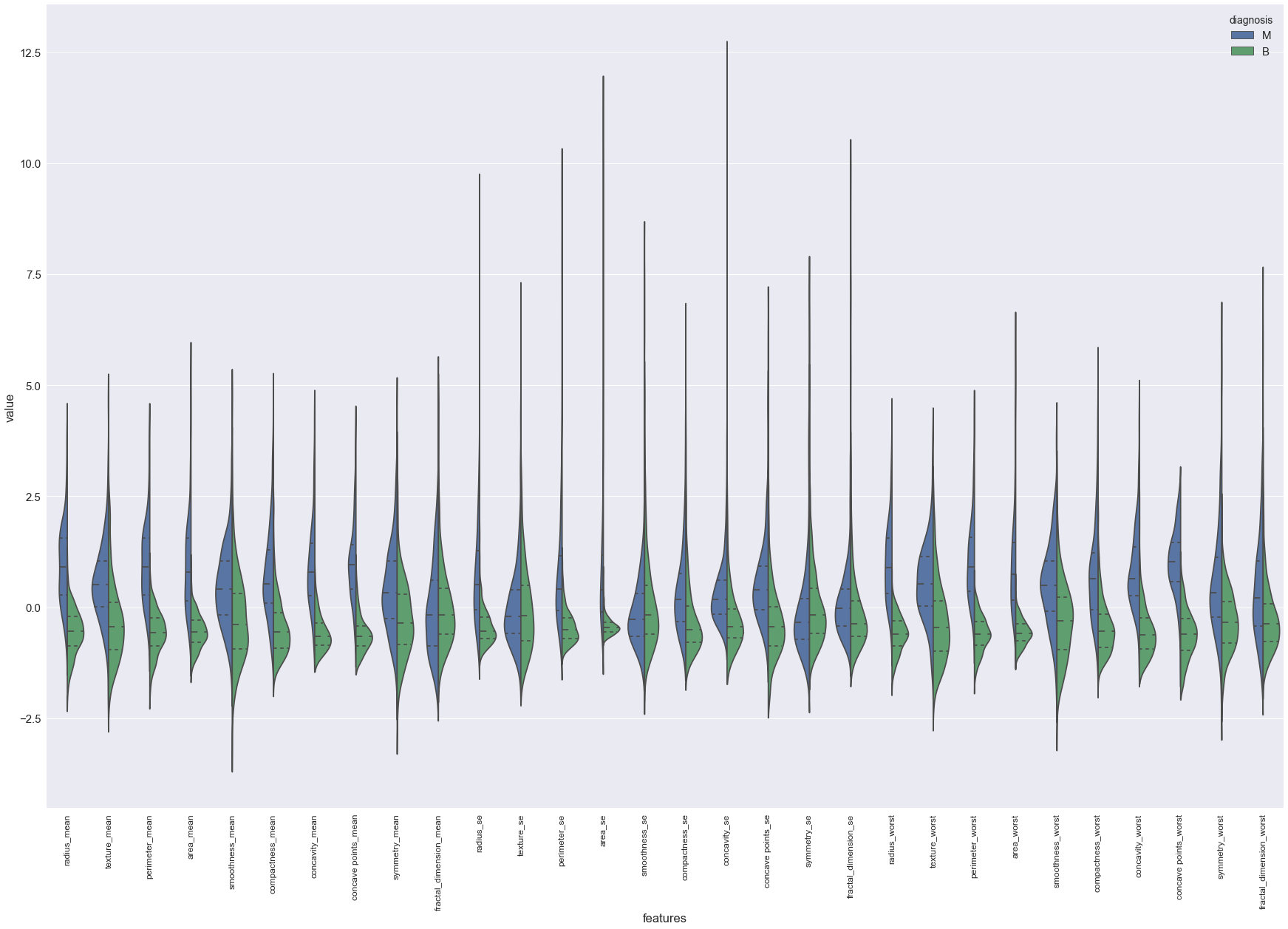Conclusion: in some features, like radius_mean, texture_mean, median of each class separated, so they can be useful for classification. Other features, like smoothness_se, are not so separated and my be less useful for classification. Most all the features have normal-like distribution with long tail.

### 3.4 Dimensionality reduction¶

Apply pca for dimensionality reduction:

In :
pca = PCA(random_state=24)
pca.fit(scaled_data)

plt.figure(figsize=(10, 10))
plt.plot(pca.explained_variance_ratio_, linewidth=2)
plt.xlabel('Number of components');
plt.ylabel('Explained variance ratio');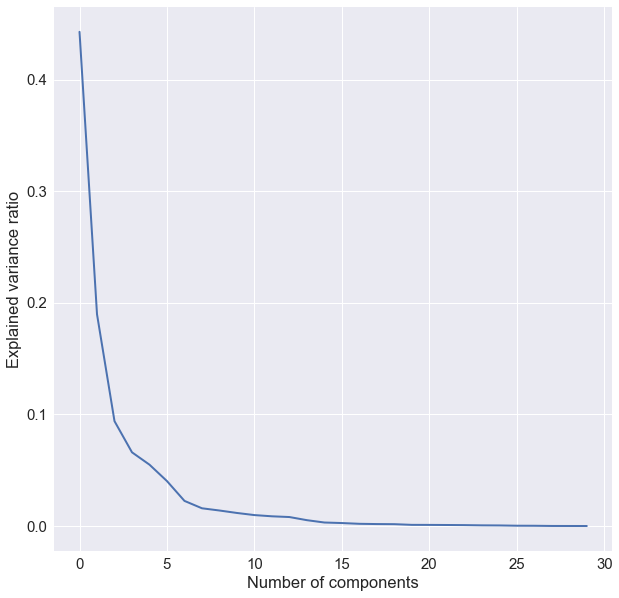Conclusion: according to elbow method 3 components may be choosen.

Check the number of components for explaining data variance:

In :
components = range(1, pca.n_components_ + 1)
plt.figure(figsize=(15, 5));
plt.bar(components, np.cumsum(pca.explained_variance_ratio_));
plt.hlines(y = .95, xmin=0, xmax=len(components), colors='green');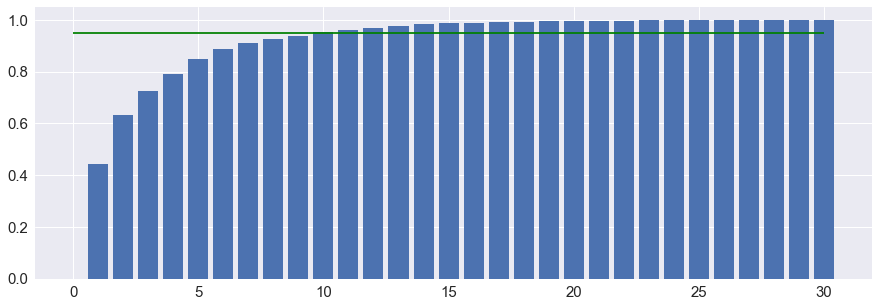Conclusion: The two first components explains the 0.6324 of the variance. We need 10 principal components to explain more than 0.95 of the variance and 17 to explain more than 0.99.

Reduce dimensions of data and plot it:

In :
pca_two_comp = PCA(n_components=2, random_state=24)
two_comp_data = pca_two_comp.fit_transform(scaled_data)
plt.scatter(x=two_comp_data[:, 0], y=two_comp_data[:, 1],
c=target['diagnosis'].map({'M': 'red', 'B': 'green'}))
plt.show()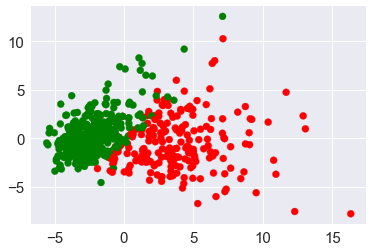Conclusion: data is good enough separable using only two components.

## 4. Insights and found dependencies¶

Data summary:

• there are a lot of groups with correlated features. Next we have to get rid from multi-collinearity by selectig one feature for each group.
• ration of examples in each class 0.67/0.27. No skewed classes here, which is important for metric selection;
• differences in features stitistics (mean) for each class are statisticalli important. So this features will be important for classification.
• there are outliers in data. It's important to get rid of them for outliers-sensetive models (logistic regression for example) before training;
• PCA shows thad data is good enough separable using only 3-5 features.

## 5. Metrics selection¶

Predict whether the cancer is benign or malignant is a binary classification task. Here we don't face the probem of skewed classes. So accuracy metric will be a good choice for model evaluation. Also this metric is simple enough, thus highly interpretable.

$$Accuracy=\frac{Number~of~corrected~predictions}{Total~number~of~predictions}$$

Aslo for the test set we will calculate precision and recall.

## 6. Model selection¶

As model was selected Logistic regression because:

• works well with non categorical features (in our data all features are continious);
• robust to small noise in the data;
• cases of multi-collinearity can be handled by implementing regularization;
• works well if there are no missing data;
• efficient implementation availavle;
• feature space of current task is not large.

## 7. Data preprocessing¶

### 7.1 Drop useless columns¶

Drop constant column Unnamed: 32 and useless folumn id for classification.

In :
X = df.drop(['id', 'Unnamed: 32', 'diagnosis'], axis=1)
y = df['diagnosis'].map(lambda x: 1 if x=='M' else 0)


### 7.3 Split data into train/test¶

Split data into train/test with proportional 0.7/0.3 which is common split for such amount of data.

In :
X_train, X_test, y_train, y_test = train_test_split(X, y, test_size=0.3, random_state=24)
print('Train size:', X_train.size)
print('Test size:', X_test.size)

Train size: 11940
Test size: 5130


### 7.2 Feature selection¶

First of all we should handle multi-collinearity. From each group of correleted features we will select only by one feature. So here columns to drop:

In :
corr_columns = ['perimeter_mean','radius_mean','compactness_mean',
'concave points_worst','compactness_se','concave points_se',
'texture_worst','area_worst',
'concavity_mean']


Drop correlated columns from train data:

In :
X_train = X_train.drop(corr_columns, axis=1)


Drop correlated columns from test data:

In :
X_test = X_test.drop(corr_columns, axis=1)


Check number of features left:

In :
print('Current number of features:', X_train.shape)

Current number of features: 15


### 7.3 Feature scaling¶

In :
scaler = StandardScaler()
X_train_scaled = scaler.fit_transform(X_train)
X_test_scaled = scaler.transform(X_test)


## 8. Cross-validation and adjustment of model hyperparameters¶

Use 3 splits because of we don't have large amount of training data and shuffle samples in random order.

In :
cv = StratifiedKFold(n_splits=3, random_state=24)


Model:

In :
model = LogisticRegression(random_state=24)


Model parameters:

In :
model_parameters = {'penalty': ['l1', 'l2'],
'C': np.linspace(.1, 1, 10)}


To find best hyperparameters we will use grid search as in is quite simple and efficient enough.

In :
grig_search = GridSearchCV(model, model_parameters, n_jobs=-1, cv=cv, scoring='accuracy')

In :
%%time
grig_search.fit(X_train_scaled, y_train);

CPU times: user 122 ms, sys: 32.4 ms, total: 155 ms
Wall time: 322 ms

Out:
GridSearchCV(cv=StratifiedKFold(n_splits=3, random_state=24, shuffle=False),
error_score='raise',
estimator=LogisticRegression(C=1.0, class_weight=None, dual=False, fit_intercept=True,
intercept_scaling=1, max_iter=100, multi_class='ovr', n_jobs=1,
penalty='l2', random_state=24, solver='liblinear', tol=0.0001,
verbose=0, warm_start=False),
fit_params=None, iid=True, n_jobs=-1,
param_grid={'penalty': ['l1', 'l2'], 'C': array([0.1, 0.2, 0.3, 0.4, 0.5, 0.6, 0.7, 0.8, 0.9, 1. ])},
pre_dispatch='2*n_jobs', refit=True, return_train_score='warn',
scoring='accuracy', verbose=0)

Best model parameters:

In :
grig_search.best_params_

Out:
{'C': 0.2, 'penalty': 'l2'}

Best cv score:

In :
print('Accuracy:', grig_search.best_score_)

Accuracy: 0.9623115577889447


## 9. Creation of new features¶

Helper function for applying map operation to data frame attributes:

In :
def apply_cat_op(data, attrs, operation, prefix):
"""
Apply one operation to data attributes.
"""
series = [data[attr].map(operation) for attr in attrs]

new_attrs = _data.columns.values

return _data, new_attrs


Creating new features based on medicine requires strong domain knowledge. So we will create them based on mathematics nature of current features. Basic approach for numerical features for regression model is to calculate squares of features in order to capture non-linear dependencies.

Square function:

In :
sq_operation = lambda x: x**2


Create squared feature for each columns and test in with model:

In :
for column in X_train.columns:
X_train_sq, sq_attr = apply_cat_op(X_train, [column], sq_operation, 'sq_')
data = pd.concat([X_train, X_train_sq], axis=1)

scaler = StandardScaler()
data_scaled = scaler.fit_transform(data)

grig_search = GridSearchCV(model, model_parameters, n_jobs=-1, cv=cv, scoring='accuracy')

grig_search.fit(data_scaled, y_train);

print('Column:', column, ' ',
'Accuracy:', grig_search.best_score_, ' ',
'Best params:', grig_search.best_params_)

Column: texture_mean   Accuracy: 0.9597989949748744   Best params: {'C': 0.1, 'penalty': 'l2'}
Column: area_mean   Accuracy: 0.9597989949748744   Best params: {'C': 0.1, 'penalty': 'l2'}
Column: smoothness_mean   Accuracy: 0.9623115577889447   Best params: {'C': 0.30000000000000004, 'penalty': 'l2'}
Column: symmetry_mean   Accuracy: 0.9597989949748744   Best params: {'C': 0.2, 'penalty': 'l2'}
Column: fractal_dimension_mean   Accuracy: 0.964824120603015   Best params: {'C': 0.2, 'penalty': 'l2'}
Column: texture_se   Accuracy: 0.9597989949748744   Best params: {'C': 0.2, 'penalty': 'l2'}
Column: area_se   Accuracy: 0.9623115577889447   Best params: {'C': 0.7000000000000001, 'penalty': 'l2'}
Column: smoothness_se   Accuracy: 0.9597989949748744   Best params: {'C': 0.1, 'penalty': 'l2'}
Column: concavity_se   Accuracy: 0.9597989949748744   Best params: {'C': 0.2, 'penalty': 'l2'}
Column: symmetry_se   Accuracy: 0.9597989949748744   Best params: {'C': 0.2, 'penalty': 'l2'}
Column: fractal_dimension_se   Accuracy: 0.9623115577889447   Best params: {'C': 0.2, 'penalty': 'l2'}
Column: smoothness_worst   Accuracy: 0.9623115577889447   Best params: {'C': 0.5, 'penalty': 'l2'}
Column: concavity_worst   Accuracy: 0.9623115577889447   Best params: {'C': 0.5, 'penalty': 'l2'}
Column: symmetry_worst   Accuracy: 0.9597989949748744   Best params: {'C': 0.2, 'penalty': 'l2'}
Column: fractal_dimension_worst   Accuracy: 0.9597989949748744   Best params: {'C': 0.2, 'penalty': 'l2'}


As we ca see squaring feature fractal_dimension_mean, gives score improving with params {'C': 0.2, 'penalty': 'l2'}

Add new feature to train data:

In :
X_train_sq, atr = apply_cat_op(X_train, ['fractal_dimension_mean'], sq_operation, 'sq_')
X_train = pd.concat([X_train, X_train_sq], axis=1)


Add new feature to test data:

In :
X_test_sq, atr = apply_cat_op(X_test, ['fractal_dimension_mean'], sq_operation, 'sq_')
X_test = pd.concat([X_test, X_test_sq], axis=1)


#### Scale the final data:¶

In :
scaler = StandardScaler()
X_train_scaled = scaler.fit_transform(X_train)
X_test_scaled = scaler.transform(X_test)


#### Train model with best parameters on all train data:¶

In :
final_model = LogisticRegression(penalty='l2', C=0.2)
final_model.fit(X_train_scaled, y_train)

Out:
LogisticRegression(C=0.2, class_weight=None, dual=False, fit_intercept=True,
intercept_scaling=1, max_iter=100, multi_class='ovr', n_jobs=1,
penalty='l2', random_state=None, solver='liblinear', tol=0.0001,
verbose=0, warm_start=False)

## 10. Plotting training and validation curves¶

### 10.1 Training curve¶

In :
def plot_learning_curve(estimator, title, X, y, ylim=None, cv=None,
n_jobs=None, train_sizes=np.linspace(.1, 1.0, 5)):
"""
Generate a simple plot of the test and training learning curve.

Parameters
----------
estimator : object type that implements the "fit" and "predict" methods
An object of that type which is cloned for each validation.

title : string
Title for the chart.

X : array-like, shape (n_samples, n_features)
Training vector, where n_samples is the number of samples and
n_features is the number of features.

y : array-like, shape (n_samples) or (n_samples, n_features), optional
Target relative to X for classification or regression;
None for unsupervised learning.

ylim : tuple, shape (ymin, ymax), optional
Defines minimum and maximum yvalues plotted.

cv : int, cross-validation generator or an iterable, optional
Determines the cross-validation splitting strategy.
Possible inputs for cv are:
- None, to use the default 3-fold cross-validation,
- integer, to specify the number of folds.
- :term:CV splitter,
- An iterable yielding (train, test) splits as arrays of indices.

For integer/None inputs, if y is binary or multiclass,
:class:StratifiedKFold used. If the estimator is not a classifier
or if y is neither binary nor multiclass, :class:KFold is used.

Refer :ref:User Guide <cross_validation> for the various
cross-validators that can be used here.

n_jobs : int or None, optional (default=None)
Number of jobs to run in parallel.
None means 1 unless in a :obj:joblib.parallel_backend context.
-1 means using all processors. See :term:Glossary <n_jobs>
for more details.

train_sizes : array-like, shape (n_ticks,), dtype float or int
Relative or absolute numbers of training examples that will be used to
generate the learning curve. If the dtype is float, it is regarded as a
fraction of the maximum size of the training set (that is determined
by the selected validation method), i.e. it has to be within (0, 1].
Otherwise it is interpreted as absolute sizes of the training sets.
Note that for classification the number of samples usually have to
be big enough to contain at least one sample from each class.
(default: np.linspace(0.1, 1.0, 5))
"""
plt.figure()
plt.title(title)
if ylim is not None:
plt.ylim(*ylim)
plt.xlabel("Training examples")
plt.ylabel("Score")
train_sizes, train_scores, test_scores = learning_curve(
estimator, X, y, cv=cv, n_jobs=n_jobs, train_sizes=train_sizes)
train_scores_mean = np.mean(train_scores, axis=1)
train_scores_std = np.std(train_scores, axis=1)
test_scores_mean = np.mean(test_scores, axis=1)
test_scores_std = np.std(test_scores, axis=1)
plt.grid()

plt.fill_between(train_sizes, train_scores_mean - train_scores_std,
train_scores_mean + train_scores_std, alpha=0.1,
color="r")
plt.fill_between(train_sizes, test_scores_mean - test_scores_std,
test_scores_mean + test_scores_std, alpha=0.1, color="g")
plt.plot(train_sizes, train_scores_mean, 'o-', color="r",
label="Training score")
plt.plot(train_sizes, test_scores_mean, 'o-', color="g",
label="Cross-validation score")

plt.legend(loc="best")
return plt

In :
plot_learning_curve(final_model, 'Logistic regression',
X_train_scaled, y_train, cv=cv);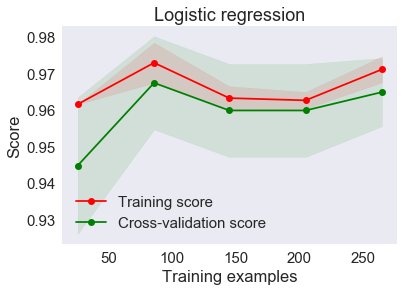Conclusion: such gap between training and validating curve indicates overfitting. But we can see that validation curve increasing with increasing amount of training examples, so more data is likely to help beat overfitting.

### 10.2 Validation curve¶

Plotting validation curve function:

In :
def plot_validation_curve(estimator, title, X, y, param_name, param_range,
cv=None, scoring=None, ylim=None, n_jobs=None):
"""
Generates a simple plot of training and validation scores for different parameter values.

Parameters
----------
estimator : object type that implements the "fit" and "predict" methods
An object of that type which is cloned for each validation.

title : string
Title for the chart.

X : array-like, shape (n_samples, n_features)
Training vector, where n_samples is the number of samples and
n_features is the number of features.

y : array-like, shape (n_samples) or (n_samples, n_features), optional
Target relative to X for classification or regression;
None for unsupervised learning.

param_name : string
Name of the parameter that will be varied.

param_range : array-like, shape (n_values,)
The values of the parameter that will be evaluated.

cv : int, cross-validation generator or an iterable, optional
Determines the cross-validation splitting strategy.
Possible inputs for cv are:
- None, to use the default 3-fold cross-validation,
- integer, to specify the number of folds.
- :term:CV splitter,
- An iterable yielding (train, test) splits as arrays of indices.

For integer/None inputs, if y is binary or multiclass,
:class:StratifiedKFold used. If the estimator is not a classifier
or if y is neither binary nor multiclass, :class:KFold is used.

Refer :ref:User Guide <cross_validation> for the various
cross-validators that can be used here.

scoring : string, callable or None, optional, default: None
A string (see model evaluation documentation) or
a scorer callable object / function with signature
scorer(estimator, X, y).

ylim : tuple, shape (ymin, ymax), optional
Defines minimum and maximum yvalues plotted.

n_jobs : int or None, optional (default=None)
Number of jobs to run in parallel.
None means 1 unless in a :obj:joblib.parallel_backend context.
-1 means using all processors. See :term:Glossary <n_jobs>
for more details.

"""
train_scores, test_scores = validation_curve(
estimator, X, y, param_name, param_range,
cv=cv, scoring=scoring, n_jobs=n_jobs)

train_scores_mean = np.mean(train_scores, axis=1)
train_scores_std = np.std(train_scores, axis=1)
test_scores_mean = np.mean(test_scores, axis=1)
test_scores_std = np.std(test_scores, axis=1)

plt.figure()
plt.grid()
plt.title(title)
plt.xlabel(param_name)
plt.ylabel("Score")
if ylim is not None:
plt.ylim(*ylim)
plt.semilogx(param_range, train_scores_mean, 'o-', label="Training score",
color="darkorange")
plt.fill_between(param_range, train_scores_mean - train_scores_std,
train_scores_mean + train_scores_std, alpha=0.2,
color="darkorange")
plt.semilogx(param_range, test_scores_mean, 'o-', label="Cross-validation score",
color="navy")
plt.fill_between(param_range, test_scores_mean - test_scores_std,
test_scores_mean + test_scores_std, alpha=0.2,
color="navy")
plt.legend(loc="best")
return plt


Plot validation curve for model complexity parameter:

In :
plot_validation_curve(final_model, 'Logistic regression', X_train_scaled, y_train,
'C', model_parameters['C'],
cv=cv, scoring='accuracy');